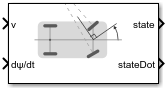# Ackermann Kinematic Model

Car-like vehicle motion using Ackermann kinematic model

• Library:
• Robotics System Toolbox / Mobile Robot Algorithms## Description

The Ackermann Kinematic Model block creates a car-like vehicle model that uses Ackermann steering. This model represents a vehicle with two axles separated by the distance, `Wheel base`. The state of the vehicle is defined as a four-element vector, [x y theta psi], with an global xy-position, vehicle heading, theta, and steering angle, psi. The vehicle heading is defined at the center of the rear axle. Angles are specified in radians. The steering input for the vehicle is given as `dpsi/dt`, in radians per second.

## Ports

### Input

expand all

Vehicle speed, specified in meters per second.

Angular velocity of the vehicle, specified in radians per second.

### Output

expand all

Current xy-position, orientation, and steering angle, specified as [x y theta psi], in meters and radians.

The linear and angular velocities of the vehicle, specified as a [xDot yDot thetaDot psiDot] vector in meters per second and radians per second. The linear and angular velocities are calculated by taking the time derivatives of the `state` output.

## Parameters

expand all

The wheel base refers to the distance between the front and rear vehicle axles, specified in meters.

The wheel speed range is a two-element vector that provides the minimum and maximum vehicle wheel speeds, [MinSpeed MaxSpeed], specified in radians per second.

The maximum steering angle, refers to the maximum amount the vehicle can be steered to the right or left, specified in radians. The default value, `pi/4` provides the vehicle with minimum turning radius, 0.

The initial x-, `y`-position, heading angle, theta, and steering angle, psi, of the vehicle.

• `Interpreted execution` — Simulate model using the MATLAB® interpreter. For more information, see Simulation Modes (Simulink).

• `Code generation` — Simulate model using generated C code. The first time you run a simulation, Simulink® generates C code for the block. The C code is reused for subsequent simulations, as long as the model does not change.

Tunable: No

 Lynch, Kevin M., and Frank C. Park. Modern Robotics: Mechanics, Planning, and Control. 1st ed. Cambridge, MA: Cambridge University Press, 2017.

##### Support평가판 신청